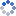加载中…MATLAB技术论坛
• 博客等级：• 博客积分：0
• 博客访问：590,608
• 关注人气：489
• 获赠金笔：0支
• 赠出金笔：0支
• 荣誉徽章：

## 【原创】绕任意空间轴旋转三维图形

(2011-07-31 10:55:19)

### 杂谈

1. function Pr=rot3d(P,origin,dirct,theta)
2. % 将坐标点P绕着，过origin点，方向为dirct的直线，旋转theta角
3. % P：需要旋转的做标集合，n×3矩阵
4. % origin：转轴通过的点，1×3向量
5. % direct：转轴方向向量，1×3向量
6. % theta：旋转角度，单位弧度
7. %
8. % By LaterComer of MATLAB技术论坛
10. % Contact me matlabsky@gmail.com
11. % Modifid at 2011-07-26 19:51:32

12. dirct=dirct(:)/norm(dirct);

13. A_hat=dirct*dirct';

14. A_star=[0,         -dirct(3),      dirct(2)
15.         dirct(3),          0,     -dirct(1)
16.        -dirct(2),   dirct(1),            0];
17. I=eye(3);
18. M=A_hat+cos(theta)*(I-A_hat)+sin(theta)*A_star;
19. origin=repmat(origin(:)',size(P,1),1);
20. Pr=(P-origin)*M'+origin;

1. clc
2. clear
3. close all

4. % 随机生成转轴通过的点
5. origin=rand(1,3)*10;
6. % 随机生成转轴方向
7. direct=rand(1,3)*10;
8. % 随机生成旋转角度
9. theta=rand*5;

10. [x,y,z]=peaks;

11. %% 图形比较
12. % 使用MATLAB自带rotate函数
13. figure
14. mesh(x,y,z);
15. hold on
16. h=mesh(x,y,z);
17. % rotate函数中角单位是角度
19. title('使用MATLAB自带rotate函数')

20. % 使用自己编写的rot3d函数
21. figure
22. mesh(x,y,z)
23. hold on
24. P=[x(:),y(:),z(:)];
25. Pr=rot3d(P,origin,direct,theta);
26. xr=reshape(Pr(:,1),size(x));
27. yr=reshape(Pr(:,2),size(x));
28. zr=reshape(Pr(:,3),size(x));
29. mesh(xr,yr,zr);
30. title('使用自己编写rot3d函数')

31. %% 数据比较
32. % 使用MATLAB自带rotate函数
33. % 由于该函数直接将图形旋转，如果想得到旋转之后的数据
34. % 此时可以使用get函数直接从图形的xdata,ydata和zdata属性中获取
35. xq=get(h,'xdata');
36. yq=get(h,'ydata');
37. zq=get(h,'zdata');

38. % 使用自己编写的rot3d函数
39. % 该函数自动返回旋转的数据而不是图形
40. % 也就是上面的xr,yr,zr

41. % 现在比较两组数据是否一致
42. disp('坐标Y的最大差距')
43. max(max(abs(yq-yr)))

44. disp('坐标X的最大差距')
45. max(max(abs(xq-xr)))

46. disp('坐标Z的最大差距')
47. max(max(abs(zq-zr)))

1. 坐标X的最大差距

2. ans =

3.      0

4. 坐标Y的最大差距

5. ans =

6.      0

7. 坐标Z的最大差距

8. ans =

9.   1.7764e-015

0